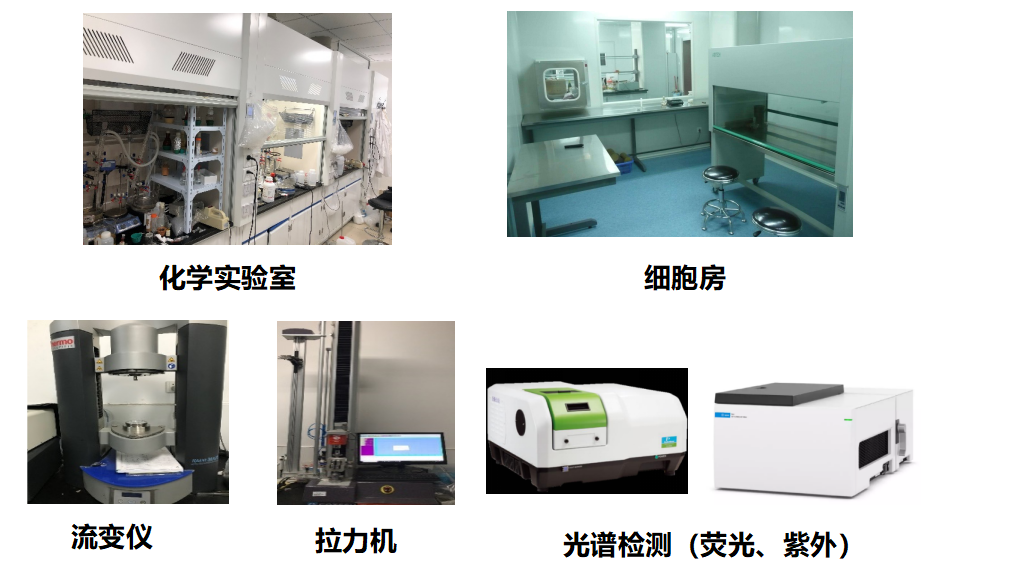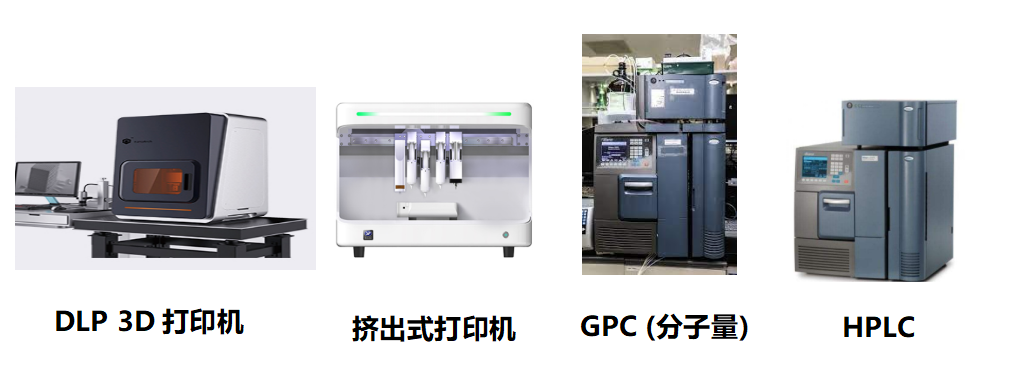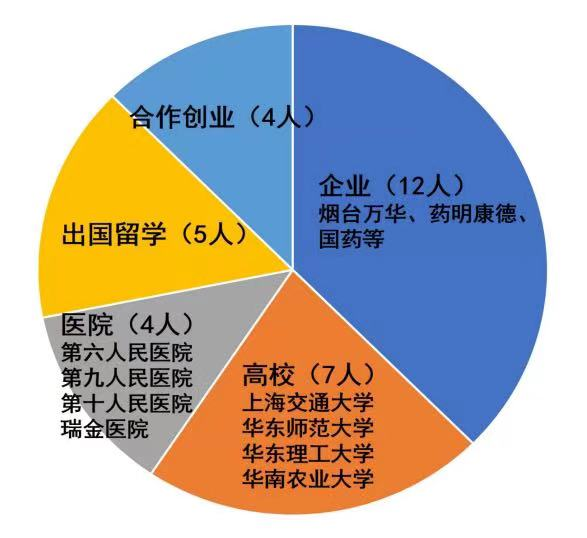01导师团队介绍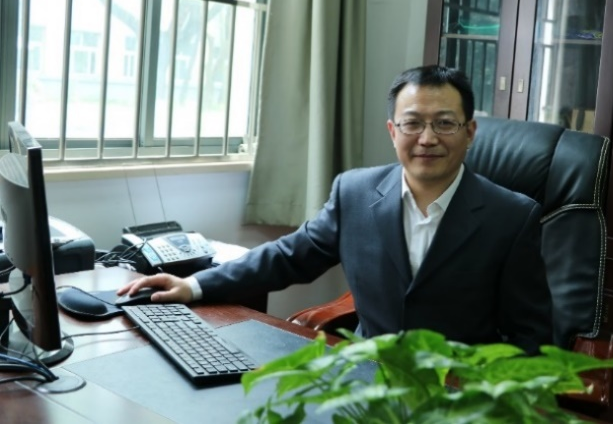• 教授 ，博士生导师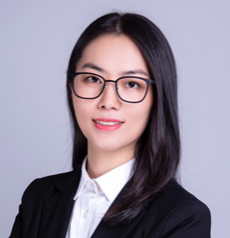• 研究员，博士生导师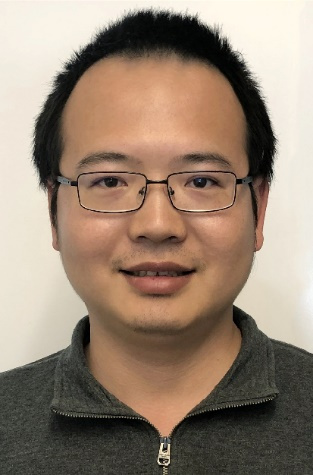• 助理研究员

02团队的师兄师姐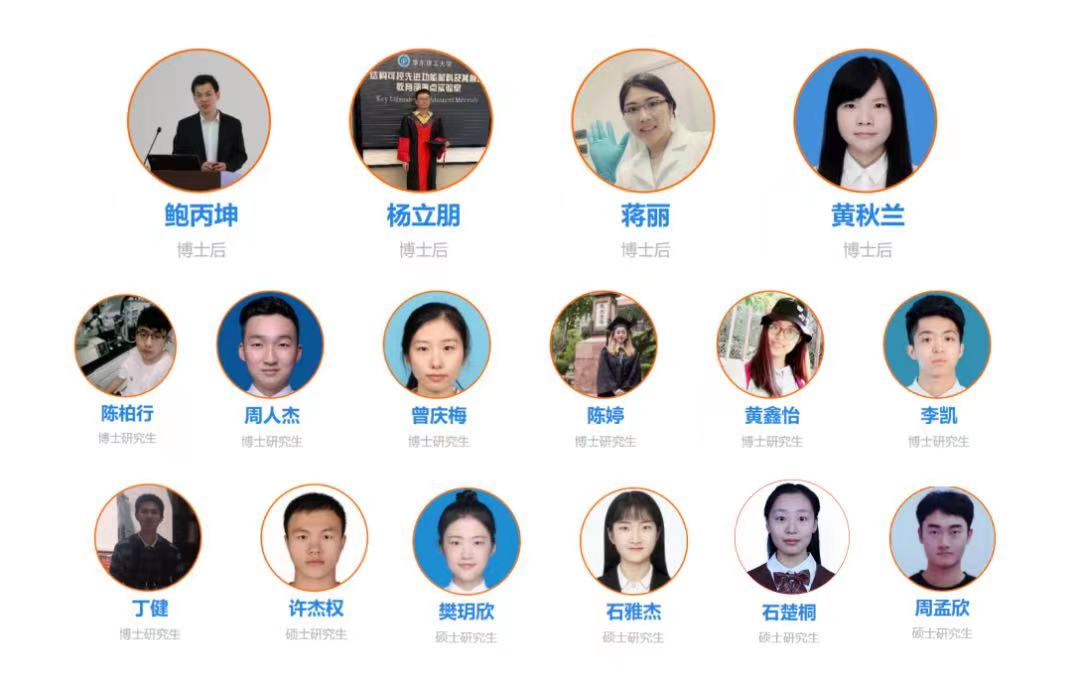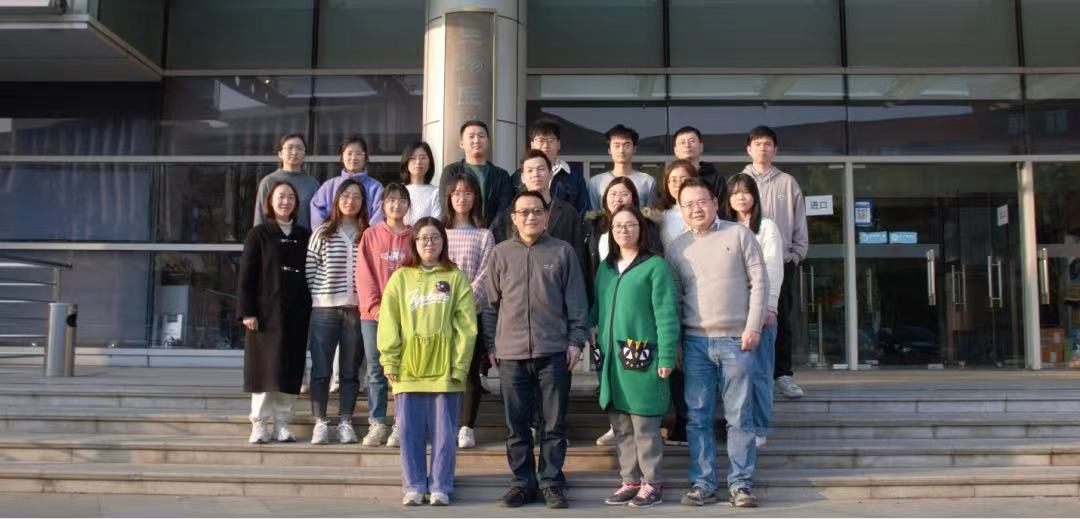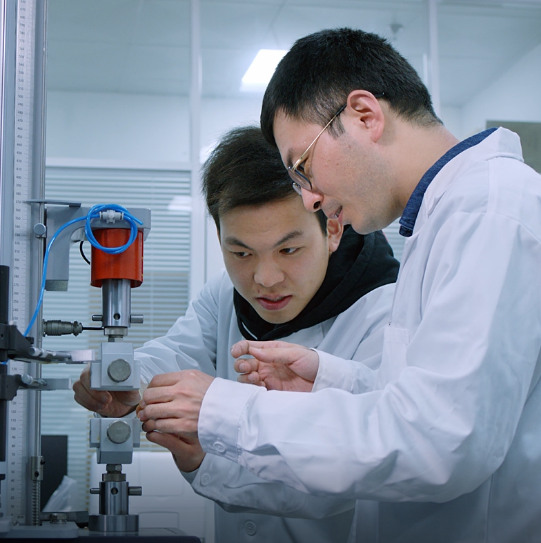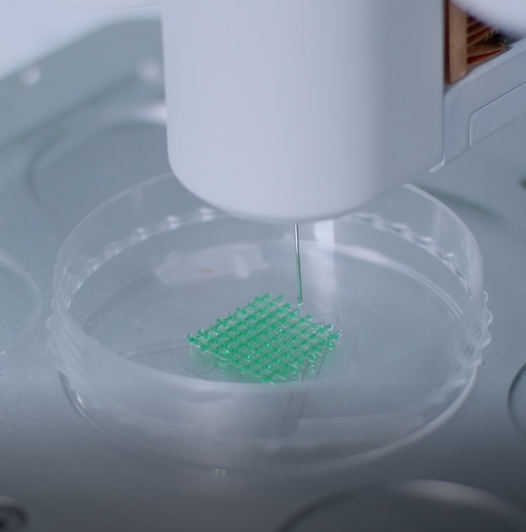03特色研究方向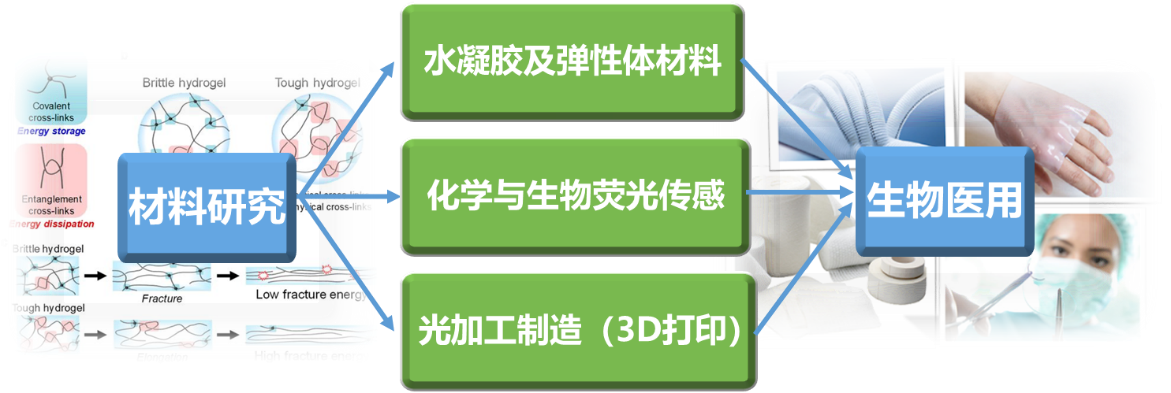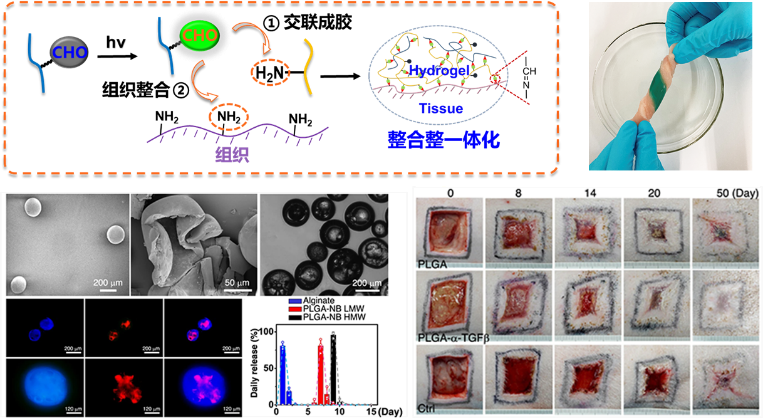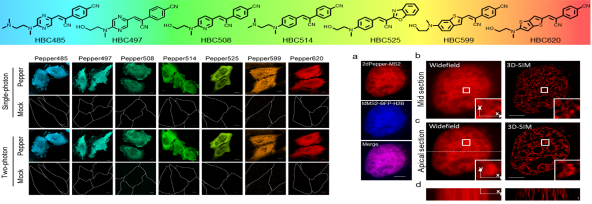04课题组近年成果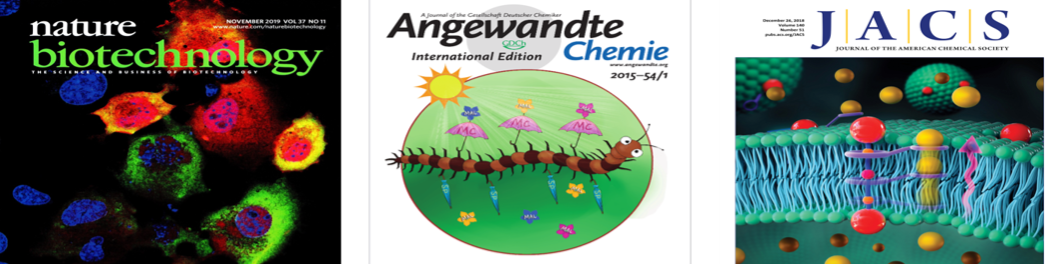1. Nat. Biotechnol. (IF =50.9), 2019, 37, 1287.

2. Nat. Chem. Biol. (IF = 12.6), 2021, 17, 1289.

3. Nat. Commun.(IF =14.9), 2021, 12, 1670.

4. Nat. Commun. (IF =14.9), 2019, 10, 2060.

5. Adv. Mater. (IF =30.1), 2021, 33, 2105667.

6. Adv. Mater.(IF =30.1), 2016, 28, 2724.

7. Adv. Mater. (IF =30.1), 2014, 26, 3912.

8. Adv. Mater. (IF =30.1), 2013, 24, 1981.

9. J. Am. Chem. Soc.(IF =15.4), 2018, 140, 17992.

10. J. Am. Chem. Soc. (IF =15.4), 2012, 134, 5052.

11. Angew. Chem. Int. Ed. (IF =15.3), 2018, 57, 3722.

12. Angew. Chem. Int. Ed. (IF =15.3), 2015, 54, 174.

05课题组近年成果